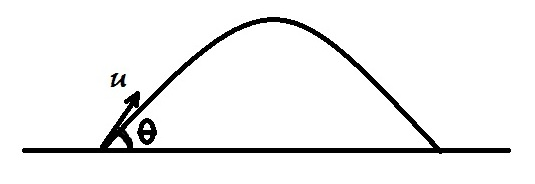# Always moving away!A projectile is launched at an angle $\theta$ with initial velocity $u$. Let $\theta = \theta_{max}$ be the largest acute angle for which the projectile is always moving away from its launch point. Determine $\lfloor\theta_{max}\rfloor$ (in degrees).

Details and Assumptions:

• $\bullet$ $g \approx 10m/s^2$
×